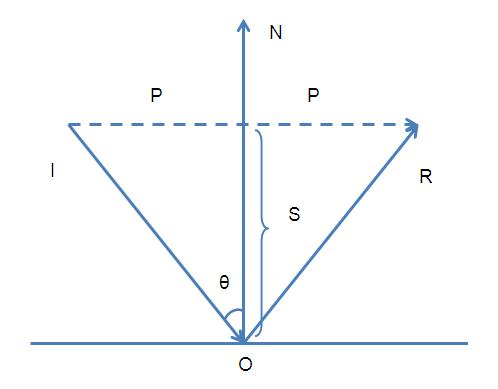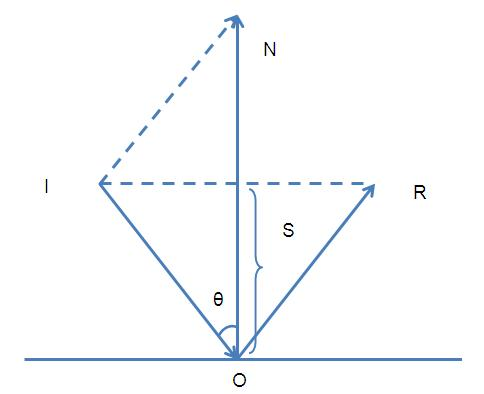# 游戏中用到物理知识（为自己）

 1 2 3 4 5 6 7 8 9 10 //Find the center of the screen             tempVector2 = new Vector3(Screen.width * 0.5f,0,Screen.height * 0.5f);             //Get mouse position             tempVector = Input.mousePosition;             //Set tempVector z to tempVector.y             tempVector.z = tempVector.y;             //Set tempVector y to 0             tempVector.y = 0;             //Set lookDir to  tempVector - tempVector2             lookDir = tempVector - tempVector2;

 1 2 //Rotate player         transform.rotation =     Quaternion.LookRotation(lookDir);

 //Get right joystick x position             float lX = rightJoystick.GetComponent().position.x;             //Get right joystick y position             float lY = rightJoystick.GetComponent().position.y; //Set lookDir x to joystick x position and dir z joystick y position                 lookDir = new Vector3(lX,0,lY); //Rotate player         transform.rotation = Quaternion.LookRotation(lookDir);

 1 2 3 // For a touchpad, let's just set the position directly based on distance from initial touchdown                     position.x = Mathf.Clamp( ( touch.position.x - fingerDownPos.x ) / ( touchZone.width / 2 ), -1, 1 );                     position.y = Mathf.Clamp( ( touch.position.y - fingerDownPos.y ) / ( touchZone.height / 2 ), -1, 1 );R = 2P - I              （1）

P = I + S               （2）ON = 2S

R = I + 2S